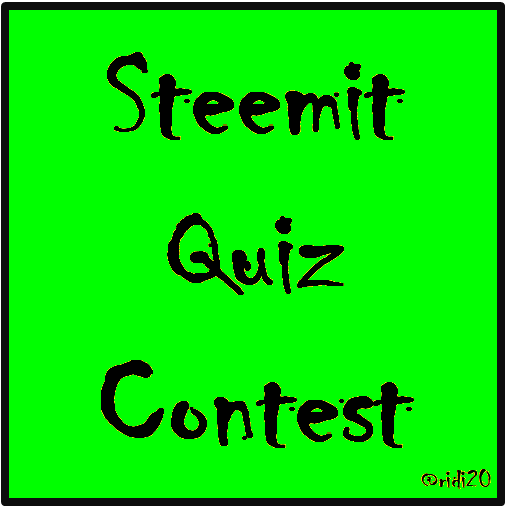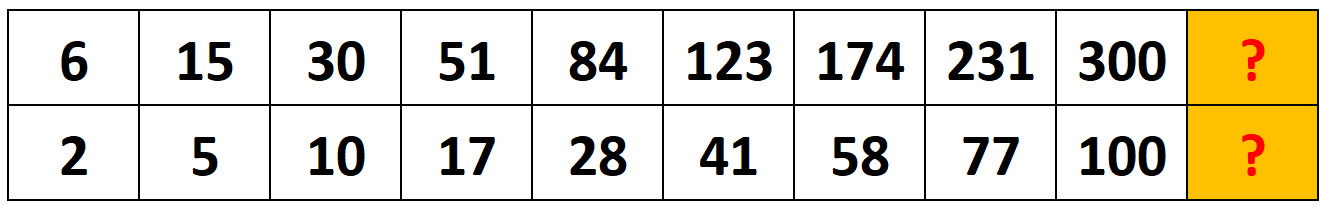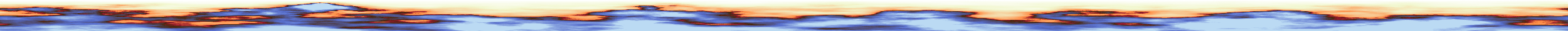# 💲10-SBD Price- ❓Steemit Quiz Contest❓ - Quiz #2 - Here we go again, have fun ✌

in #contest4 years ago# Here we go with the next quiz, have fun!# What are the next two numbers?# The quiz will be resolved the next day.# This is how it works:

### ❗❗You can only comment once and editing your comment is forbidden❗❗# 👉 Upvote this post 👈

(applies for all steemit user! If you want to get the point, you have to upvote this post)

# 👉 Resteem this post 👈

(applies only to user, who did not resteem this contest so far. One resteem is enough!)

# 👉 And of course comment the right answer 👈

Sort:

Hmm, my results were 375 and 125
I saw this pattern of
'+4' '+2' '+2' and '+2',
which lead me finally to the result of 125.
If we multiply the 125 with 3,
like the results before,
we get the result of 375.Legend:
Red color = Absolute Relevant
Blue color = Extra information, semi-relevant

387 and 129

man you are quick

I was lucky enough to see it 1 minute after it was posted.

The bottom row is increasing by these numbers:
3, 5, 7, 11, 13, 17, 19, 23... and then a ???
What do these numbers have in common? They are prime numbers! And the next prime number after 23, is the number 29. So the bottom ??? is 129.

The top row equals the bottom row times 3, so 129*3 = 387

so it's 387 and 129

387 just multiply number bellow by 3
129 is 100+ next number that can only be divided by itself... 19...23...29

Top is 387 and bottom is 129